# 1. List Comprehensions

Anything that can be done with a for-loop can be done as a comprehension.

## 1.1. List comprehension

List comprehensions provide a concise way to create lists. They are more readable than other approaches.
They are used to make new lists where each element is the result of some operations applied to each member of another sequence or iterable.
They can create a subsequence of those elements that satisfy a certain condition.

Syntax:

new_list = [expression for item in iterable]
Parameters:
• expression – the item variable only (e.g. x) or any expression such as one that uses the item variable (e.g. x * x).

• item – a variable.

• iterable – iterable objects like strings, lists, dictionaries, range function and others.

A list comprehension consists of brackets containing an expression followed by a for clause.
The result will be a new list created by evaluating the expression in the context of the for clause.

The first 2 examples illustrate simple list comprehensions without doing anything with the values.

### 1.1.1. List Example: list

In the code below, a for-loop is used to get each value from an original list then append it to a new list, my_list.
```nums = [1, 3, 6, 10, 15, 21, 28]

# I want 'n' for each 'n' in nums
my_list = []
for n in nums:
my_list.append(n)
print(my_list)
```
The printed list is: [1, 3, 6, 10, 15, 21, 28]
The list comprehension, `my_list_comprehension = [n for n in nums]`, does this in one line.
```nums = [1, 3, 6, 10, 15, 21, 28]

my_list_comprehension = [n for n in nums]
print(my_list_comprehension)
```
The diagram below has colouring to compare the use of for-loops with comprehensions.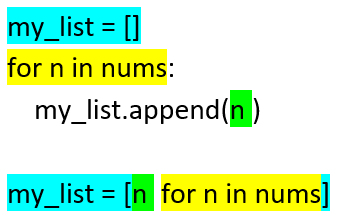### 1.1.2. List Example: range

In the code below, a for-loop is used to get each value from the range function then append it to a new list, my_list.
```# I want 'n' for each 'n' from 1 to 10
my_list = []
for n in range(1, 11):
my_list.append(n)
print(my_list)
```
The printed list is: [1, 2, 3, 4, 5, 6, 7, 8, 9, 10]
The list comprehension, `my_list_comprehension = [n for n in range(1, 11)]`, does this in one line.
```my_list_comprehension = [n for n in range(1, 11)]
print(my_list_comprehension)
```

### 1.1.3. List Example: n * n

In the code below, a for-loop is used to get each value from an original list then append a calculated valu, n * n, to a new list, my_list.
```nums = [1, 3, 6, 10, 15, 21, 28]

# I want 'n*n' for each 'n' in nums
my_list = []
for n in nums:
my_list.append(n * n)
print(my_list)
```
The printed list is: [1, 9, 36, 100, 225, 441, 784]
The list comprehension, `my_list_comprehension = [n * n for n in nums]`, does this in one line.
```nums = [1, 3, 6, 10, 15, 21, 28]

my_list_comprehension = [n * n for n in nums]
print(my_list_comprehension)
```
The diagram below has colouring to compare the use of for-loops with comprehensions.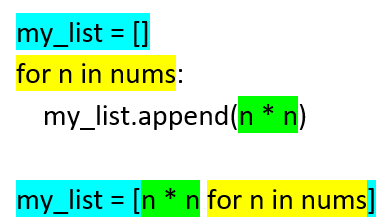### 1.1.4. Practice Questions

1. Use a list comprehension to create a list of 2 * n for each n in [0, 1, 1, 2, 3, 5, 8].

2. Use a list comprehension to create a list of 2 * n - 1 for each n in [2, 4, 6, 8, 10].

3. Use a list comprehension to create a list of n ** 3 for each n in [1, 2, 3, 4].

## 1.2. List comprehension: conditions

Syntax:

new_list = [expression for item in iterable if condition == True]
Parameters:
• expression – the item variable only (e.g. x) or any expression such as one that uses the item variable (e.g. x*x, x.upper()).

• item – variable.

• iterable – iterable objects like strings, lists, dictionaries, range function and others.

• condition – any condition.

A list comprehension consists of brackets containing an expression followed by a for clause, then zero or more for or if clauses.
The result will be a new list resulting from evaluating the expression in the context of the for and if clauses which follow it.
e.g newlist = [2 * n for n in range(5) if n % 2 = 0]

### 1.2.1. List Example: Modulo

In the code below, a for-loop is used to get a list of event numbers from a list.
The modulo, `n % 2`, returns 0 for even numbers.
```nums = [1, 3, 6, 10, 15, 21, 28]

# I want n for each n in nums if n is even
my_list = []
for n in nums:
if n % 2 == 0:
my_list.append(n)
print(my_list)
```
The printed list is: [6, 10, 28]
The list comprehension, `my_list_comprehension = [n for n in nums if n % 2 == 0]`, does this in one line.
```nums = [1, 3, 6, 10, 15, 21, 28]

# I want n for each n in nums if n is even
my_list_comprehension = [n for n in nums if n % 2 == 0]
print(my_list_comprehension)
```
The diagram below has colouring to compare the use of for-loops with comprehensions.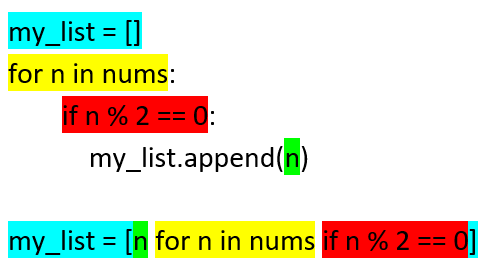### 1.2.2. Practice Questions

1. Use a list comprehension to create a list of even n for each n in [1, 2, 3, 5, 8].

2. Use a list comprehension to create a list of odd n for each n in [1, 2, 3, 5, 8].

## 1.3. List comprehension: nested

Syntax:

new_list = [expression for item1 in iterable1 for item2 in iterable2]
Parameters:
• expression – an expression using item1 and item2.

• item2 – variable for iterable1.

• iterable1 – iterable objects like strings, lists, dictionaries, range function and others.

• item2 – variable for iterable2.

• iterable2 – a second iterable objects like strings, lists, dictionaries, range function and others.

### 1.3.1. List Example: grid coordinates

In the code below, a nested for-loop is used to get a list of grid coordinates.
Each grid coordinate is a tuple. e.g. (‘A’, 1)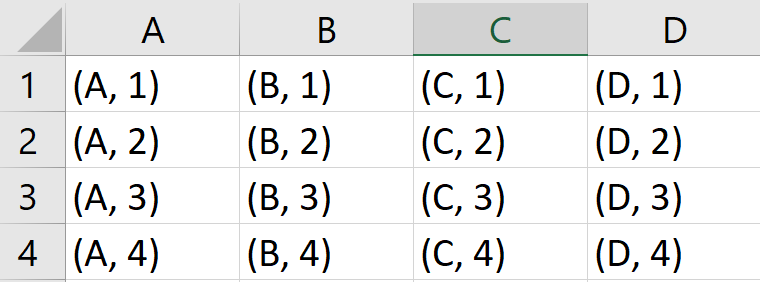The output will be: [(‘A’, 1), (‘A’, 2), (‘A’, 3), (‘A’, 4), (‘B’, 1), (‘B’, 2), (‘B’, 3), (‘B’, 4), (‘C’, 1), (‘C’, 2), (‘C’, 3), (‘C’, 4), (‘D’, 1), (‘D’, 2), (‘D’, 3), (‘D’, 4)]
```# I want a (letter, num) pair for each letter in 'ABCD' and each number in '1234'
my_list = []
for letter in "ABCD":
for num in range(1, 5):
my_list.append((letter, num))
print(my_list)
```
The list comprehension does the nested for-loop in one line.
```my_list_comprehension = [(letter, num) for letter in "ABCD" for num in range(1, 5)]
print(my_list_comprehension)
```
The diagram has colouring to compare the use of for-loops with comprehensions.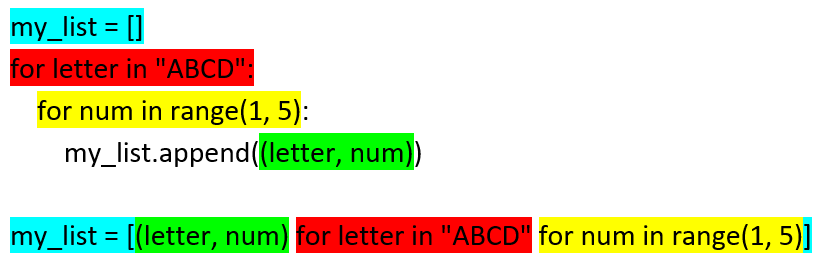### 1.3.2. Practice Questions

1. Starting with two lists, [‘A’, ‘B’], [‘X’, ‘Y’], use a list comprehension to create the list: [(‘A’, ‘X’), (‘A’, ‘Y’), (‘B’, ‘X’), (‘B’, ‘Y’)].

2. Starting with two lists, [‘A’, ‘B’], [‘X’, ‘Y’], use a list comprehension to create the list: [‘AvX’, ‘AvY’, ‘BvX’, ‘BvY’].

### 1.3.3. Summary Examples

```my_list_comprehension = [n for n in nums]
my_list_comprehension = [n for n in range(1, 11)]
my_list_comprehension = [n * n for n in nums]
my_list_comprehension = [n for n in nums if n % 2 == 0]
my_list_comprehension = [(letter, num) for letter in "ABCD" for num in range(1, 5)]
```

Set Comprehension on a List primes = [2, 2, 2, 3, 3, 5, 5, 5, 7, 11, 11, 13, 13, 13, 13] primes_squared = {p*p for p in primes} print(primes_squared)

More Complex Expressions: quadratic transformation Any expression is allowed. More complex expressions can be put in parentheses. Here, quadratic equation: 2x^2 + 5x + 10

transformed = {(2*x*x + 5*x + 10) for x in primes} print(transformed)

### 1.3.4. Making a 2D aray from a list

A list will be split up into a lists of lists, simulating a 2D array.
In the code below, a list is organised into a 2d array with x elements in each row.
[1, 3, 5, 7, 9, 11] will become [[1, 3, 5], [7, 9, 11]].
The first loop, `for i in range(0, len(nums), x)`, becomes `for i in range(0, 6, 3)` which has i values of 0 and 3.
The second loop, `for j in range(i,i+x)`, becomes `for j in range(0,3)` when i is 0, and `for j in range(3,6)` when i is 3
```nums = [1, 3, 5, 7, 9, 11]
x = 3

list_2d = []
for i in range(0, len(nums), x):
rowlist = []
for j in range(i,i+x):
rowlist.append(nums[j])
list_2d.append(rowlist)
print(list_2d)
# [[1, 3, 5], [7, 9, 11]]
```
The code below achieves the same result in a list comprehension.
```nums = [1, 3, 5, 7, 9, 11]
x = 3
list_2d = [nums[i:i+x] for i in range(0, len(nums), x)]
print(list_2d)
# [[1, 3, 5], [7, 9, 11]]
```